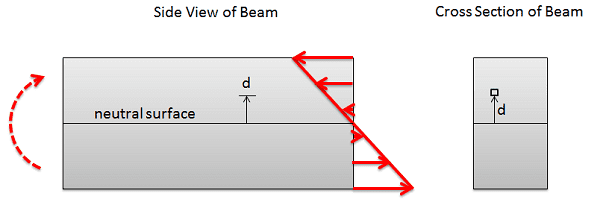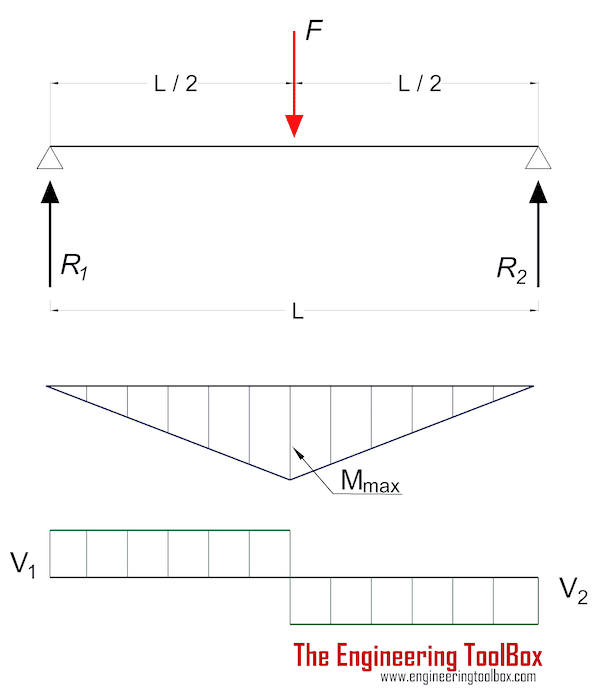# Structures: Bending moments

Summary:
Moment equilibrium
I have a quesrion about forming the eqaution of moment equilibrium.
As shown in the picture at cut 1 there are 2 vertical forces and a bending moment. I expect V=A.
If I apply moment equilibrium about the point where the bending moment is, we get that Ay*x = M(1). But how about if I take moment about point A, is this equation of moment equilibrium valid: (V*x) -M = 0(2). Until now every exercise solved I was applying moment equiibirum about the point where the bending moment is.
Now I am doing buckling and I saw an example with fixed end column. The method of section was used again and at the one end (A) there was a horizontal force(P) with fixed-end moment(Q) and on the other end (B, the sectioned end) there was an equal and opposite horizontal force (P) and bending moment M(x). The vertical distance between the two ends was v(x) and the equation for moment equilibrium was P*v(x) = M(x) + Q and I got confused about which point the moment were takend ( A or B).

#### Attachments

•Screenshot_2020-03-05 4 3 Determinate Beam Analysis learnaboutstructures com(1).png
18.6 KB · Views: 97

PhanthomJay
Homework Helper
Gold Member
Summary:: Moment equilibrium

I have a quesrion about forming the eqaution of moment equilibrium.
As shown in the picture at cut 1 there are 2 vertical forces and a bending moment. I expect V=A.
If I apply moment equilibrium about the point where the bending moment is, we get that Ay*x = M(1). But how about if I take moment about point A, is this equation of moment equilibrium valid: (V*x) -M = 0(2). Until now every exercise solved I was applying moment equiibirum about the point where the bending moment is.
you can take the sum of moments about any point equals 0 for equilibrium problems. You will note that you correctly identified V=A, so your equations 1 and 2 are exactly the same. It is often best to sum moments about a point where there are loads given or unknown, to make the solution easier.
Now I am doing buckling and I saw an example with fixed end column. The method of section was used again and at the one end (A) there was a horizontal force(P) with fixed-end moment(Q) and on the other end (B, the sectioned end) there was an equal and opposite horizontal force (P) and bending moment M(x). The vertical distance between the two ends was v(x) and the equation for moment equilibrium was P*v(x) = M(x) + Q and I got confused about which point the moment were takend ( A or B).
it would be good to see a sketch of this problem. If there is no vertical load it is not a buckling case. But still you can take moments about any point.....your equation is better written as P*v(x) - M(x) - Q = 0. Taken about the other end, it’s +P*v(x) - Q - M(x) = 0, which is the exact same result. Show a sketch to check out your plus and minus signs.

Lnewqban
Gold Member
Summary:: Moment equilibrium

I have a quesrion about forming the eqaution of moment equilibrium.
As shown in the picture at cut 1 there are 2 vertical forces and a bending moment. I expect V=A.
If I apply moment equilibrium about the point where the bending moment is, we get that Ay*x = M(1). But how about if I take moment about point A, is this equation of moment equilibrium valid: (V*x) -M = 0(2). Until now every exercise solved I was applying moment equiibirum about the point where the bending moment is.
...
It is important that you clearly understand the purpose of this confusing mathematical exercise, for this simple case and for more complex ones.
We are doing this because engineers need to determine whether or not a structural member will be able to withstand an applied load without failure or plastic deformation.

The field of strength of materials deals with forces and deformations that result from stress acting on a material.
The reason we select and analyze stresses on imaginary cross sections of a column or beam or shaft is this: natural forces of attraction and repulsion among molecules on both faces of any imaginary cross section should not be overwhelmed by the imposed external stress (all the molecules should hold together).

Any load on a structural member ends up becoming compressive stress, tensile stress, shear stress or a combination of those applied perpendicularly to or on the same plane of a cross section of that member.
We can describe any of those stresses as force or as pressure, according to the size of the area of the cross section we want to consider.

Bending moments do not exist, they are just a concept: nature only offers us a force and a lever.
Cross sections are very small levers when compared to the span of a beam or the height of a column; hence, by the law of levers, applied external forces on bending beams can become huge forces perpendicularly applied to cross sections of that beam.

There are typical graphs for many combinations of type of loads and structural members.
For simple cases like this problem, we can overlap those graphs and have an idea about what zones of the beam will be standing the greatest combination of the three types of stresses: that will be our weakest link to focus on.
Our task is to determine how the strength of the material in there compares to the magnitudes of those stresses.

Then, we take an imaginary hacksaw and make a transverse cut in that zone of the beam.
Like we must do when we replace a flat tire in our car, installing a jack is necessary to temporarily support the load that the removed wheel was supporting, so the car remains balanced.
Our imaginary jacks are imaginary forces and moments that we temporarily apply to the freshly cut cross section, in such a way that they effectively become a substitute of the missing part of the cut beam.

We can now calculate the magnitudes of those forces (compression, tension and shear) and moments that keep in perfect static balance the part of the beam that we have kept to study.
If you have been able to follow this crazy explanation this far, you could understand that there is no possibility of any moment being stand by point A in your diagram (since it is a theoretical hinge) and that the magnitude of the moment that the cut cross section is "feeling" is small ##(Force~A_y~*~lever_x)## and decreasing towards point A.

For a formal explanation, please see: